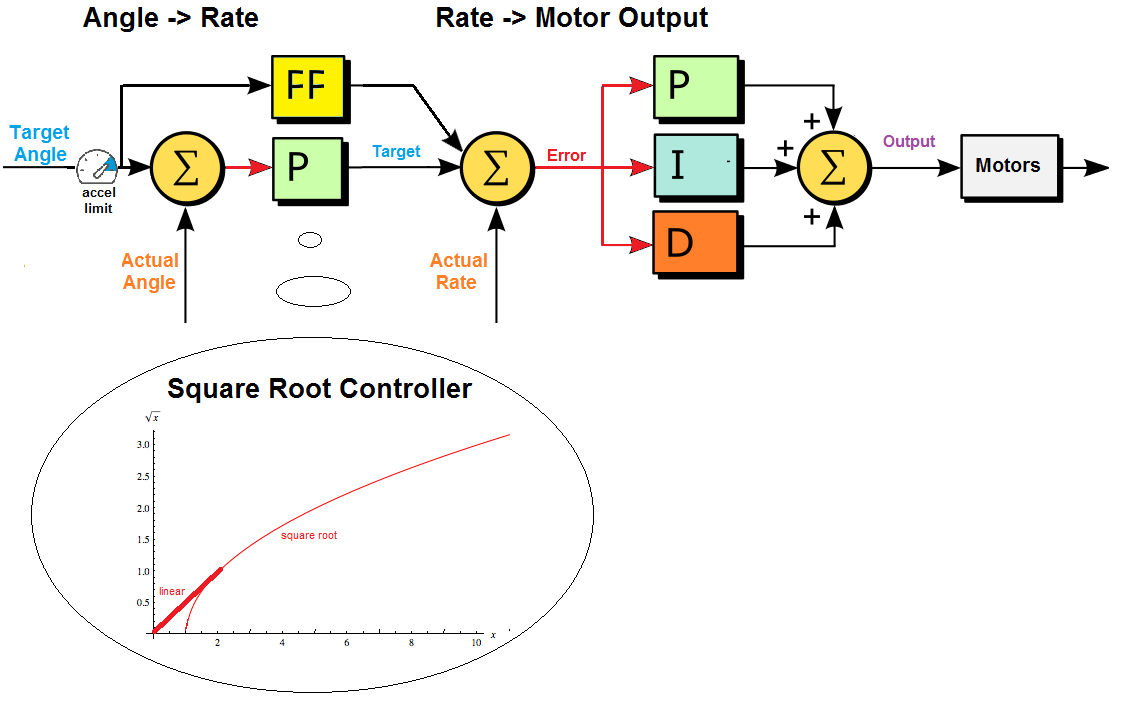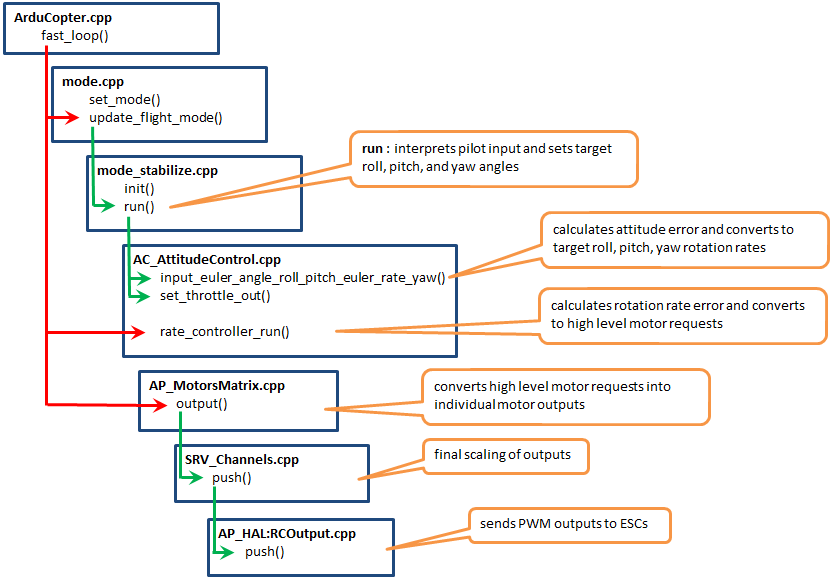# Copter Attitude Control¶

Below is a high level diagram showing how the attitude control is done for each axis. The control is done using a P controller to convert the angle error (the difference between the target angle and actual angle) into a desired rotation rate followed by a PID controller to convert the rotate rate error into a high level motor command. The “square root controller” portion of the diagram shows the curved used with the angle control’s P controller.The diagram below shows the code path followed from pilot input down to pwm output.On every update (i.e. 400hz on Pixhawk, 100hz on APM2.x) the following happens:

• the top level flight-mode.cpp’s “update_flight_mode()” function is called. This function checks the vehicle’s flight mode (ie. “control_mode” variable) and then calls the appropriate <flight mode>_run() function (i.e. stabilize_run for stabilize mode, rtl_run for RTL mode, etc). The <flight mode>_run() function can be found in the appropriately named control_<flight mode>.cpp file (i.e. control_stabilize.cpp, control_rtl.cpp, etc).
• the <flight mode>_run function is responsible for converting the user’s input (found in g.rc_1.control_in, g.rc_2.control_in, etc) into a lean angle, rotation rate, climb rate, etc that is appropriate for this flight mode. For example AltHold converts the user’s roll and pitch input into lean angles (in degrees), the yaw input is converted into a rotation rate (in degrees per second) and the throttle input is converted to a climb rate (in cm/s).
• the last thing the <flight mode>_run function must do is pass these desired angles, rates etc into Attitude Control and/or Position Control libraries (these are both held in the AC_AttitudeControl folder).
• The AC_AttitudeControl library provides 5 possible ways to control the attitude of the vehicle, the most common 3 are described below.
• angle_ef_roll_pitch_rate_ef_yaw() : this accepts an “earth frame” angle for roll and pitch, and an “earth frame” rate for yaw. For example providing this function roll = -1000, pitch = -1500, yaw = 500 means lean the vehicle left to 10degrees, pitch forward to 15degrees and rotate right at 5deg/second.
• angle_ef_roll_pitch_yaw() : this accepts “earth frame” angles for roll, pitch and yaw. similar to above except providing yaw of 500 means rotate the vehicle to 5 degrees east of north.
• rate_bf_roll_pitch_yaw() : this accepts a “body frame” rate (in degrees/sec) for roll pitch and yaw. For example providing this function roll = -1000, pitch = -1500, yaw = 500 would lead to the vehicle rolling left at 10deg/sec, pitching forward at 15deg/sec and rotating about the vehicle’s z axis at 5 deg/sec.

After any calls to these functions are made the AC_AttitudeControl::rate_controller_run() is called. This converts the output from the methods listed above into roll, pitch and yaw inputs which are sent to the AP_Motors library via it’s set_roll, set_pitch, set_yaw and set_throttle methods.

• The AC_PosControl library allows 3D position control of the vehicle. Normally only the simpler Z-axis (i.e. altitude control) methods are used because more complicated 3D position flight modes (i.e. Loiter) make use of the AC_WPNav library. In any case, some commonly used methods of this library include:

If any methods in AC_PosControl are called then the flight mode code must also call the AC_PosControl::update_z_controller() method. This will run the z-axis position control PID loops and send low-level throttle level to the AP_Motors library. If any xy-axis methods are called then AC_PosControl::update_xy_controller() must be called.

• The AP_Motors library holds the “motor mixing” code. This code is responsible for converting the roll, pitch, yaw and throttle values received from the AC_AttitudeControl and AC_PosControl libraries into absolute motor outputs (i.e. PWM values). So the higher level libs would make use of these functions:
• set_roll(), set_pitch(), set_yaw() : accepts roll, pitch and yaw values in the range of -4500 ~ 4500. These are not desired angles or even rates but rather just a value. For example set_roll(-4500) would mean roll left as fast as possible.
• set_throttle() : accepts an absolute throttle value in the range of 0 ~ 1000. 0 = motors off, 1000 = full throttle.
• There are different classes for each frame type (quad, Y6, traditional helicopter) but in each there is an “output_armed” function which is responsible for implementing the conversion of these roll, pitch, yaw and throttle values into pwm outputs. This conversion often includes implementing a “stability patch” which handles prioritising one axis of control over another when the input requests are outside the physical limits of the frame (i.e. max throttle and max roll is not possible with a quad because some motors must be less than others to cause a roll). At the bottom of the “output_armed” function there is a call to the hal.rcout->write() which passes the desired pwm values to the AP_HAL layer.
• The AP_HAL libraries (hardware abstraction layer) provides a consistent interface for all boards. In particular the hal.rc_out_write() function will cause the specified PWM received from the AP_Motors class to appear on the appropriate pwm pin out for the board.